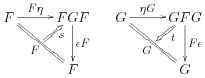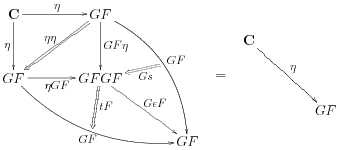Contents

# Contents

## Idea

$\phi_{A,B} : D(F A,B) \cong C(A,G B).$

A strict 2-adjunction is analogous, where $C,D$ are instead strict 2-categories, $F,G$ are strict 2-functors, and $\phi$ is a strictly 2-natural isomorphism of hom-categories, while a biadjunction or pseudoadjunction allows all of these to be “weak up to isomorphism”.

A lax 2-adjunction is instead given by a (suitably natural) pair of functors

$\hat\phi_{A,B} : D(F A,B) \overset{\to}{\leftarrow} C(A,G B) : \check\phi_{A,B}$

such that we have an adjunction $\check\phi\dashv\hat\phi$. Independently of this laxness aspect, we can make a choice of weakness or strictness for the categories, functors, and natural transformations involved; thus one might speak of “lax biadjunctions”, “lax pseudoadjunctions”, and so on.

It is not entirely clear that the term “lax 2-adjunction” is appropriate; in particular it is not an instance of the general notion of lax algebras, unlike nearly all other usages of the word “lax”. Other terms that have been used for the same or similar notions are “transcendental quasi-adjunction” (Gray) and “local adjunction” (Betti-Power).

## Definition

Just as the notion of adjunction makes sense not just in $Cat$ but in any 2-category, lax 2-adjunctions can be defined in any (possibly weak) 3-category when the above is suitably reformulated by using the 2-categorical Yoneda lemma. So, given 0-cells $C,D$ and 1-cells $F:C\to D$, $G:D\to C$ in any 3-category, we say $F$ is lax left adjoint to $G$ if we have

• 2-cells $\eta:C\Rightarrow G F$ and $\epsilon:F G \Rightarrow D$, and

• instead of strict triangle equalities, 3-cells (called triangulators) $s,t$satisfying the swallowtail identities:and(where if the 3-category is weak then coherences might need to be inserted to make these make sense.

When interpreted the a strict 3-category $2Cat$, the Gray-category $Gray$, or the tricategory $Bicat$, this yields three levels of weakness of “lax 2-adjunction” according to whether the categories, functors, and/or transformations are strict or pseudo.

## Further generalizations

The following remarks are incomplete.

One can additionally generalize to allow $\eta$ and $\epsilon$ to be lax natural transformations. Gray’s original notion of “transcendental quasi-adjunction” did this, with the 2-categories and 2-functors being strict. Lax transformations do not live in any 3-category as usually understood, but Gray’s particular case can be done in the “lax Gray-category” $Gray_\ell$.

Note also that there is no Yoneda lemma for lax transformations (though there is for pseudo ones). So in order for $\hat\phi$ to be determined by $\eta$, $\hat\phi$ must be pseudo natural in $B$, and likewise for $\check\phi$ to be determined by $\epsilon$, it must be pseudo natural in $A$. My guess is that laxity in the remaining variable will correspond to laxity of $\eta$ and $\epsilon$, and $s,t$ will come from the adjunction $\check\phi\dashv\hat\phi$. Another option is to require $\eta$ and $\epsilon$ to be pseudo natural on wide subcategories that include their components; see lax F-adjunction for an abstract context for such a definition.

One might additionally consider allowing the functors to be lax, as in (Betti-Power) (Seely). But lax functors are even harder to incorporate in a 3-category-like structure than lax transformations, since one can’t even whisker any sort of transformation by a lax functor. Seely also seems to say that $\hat\phi$ and $\check\phi$ are both strict in their first coordinate, rather than one in the first and one in the second as seems (to me) to be necessary for a Yoneda restatement.

• Renato Betti and John Power, On Local Adjointness of Distributive Bicategories
• Seely, Modelling computations: a 2-categorical framework, pdf